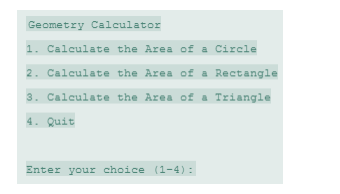# Module 08 Post-Assessment Part 2 Geometry Calculator Solved

20.00 \$

Category:
Click Category Button to View Your Next Assignment | Homework

You'll get a: . ` zip` file solution, download link after Payment

## Description

Design a Geometry class with the following methods:

 A static method that accepts the radius of a circle and returns the area of the circle. Use the following formula: Area=πr2 Use Math.PI for π and the radius of the circle for r.

 A static method that accepts the length and width of a rectangle and returns the area of the rectangle. Use the following formula: Area=Length×Width

 A static method that accepts the length of a triangle’s base and the triangle’s height. The method should return the area of the triangle.

Use the following formula: Area=Base×Height×0.5 The methods should display an error message if negative values are used for the circle’s radius, the rectangle’s length or width, or the triangle’s base or height.

Next, write a program to test the class, which displays the following menu and responds to the user’s selection:Display an error message if the user enters a number outside the range of 1 through 4 when selecting an item from the menu.